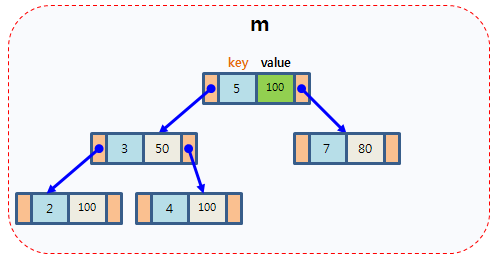New update is available. Click here to update.

# Hash Table vs STL Map

## Introduction

Hash tables and STL maps (See Maps in C++) are very useful data structures in computer science. Here we will see the comparison between their properties as well as implementation. First, we will discuss their properties one by one, and then we will differentiate them based on their key characteristics.

## Hash Table

Hash Table is a special type of data structure that stores data(key-value pair) in an associative manner and uses a hash function that maps a given value with a key to access the elements faster.

Example

In the above diagram, keys are mapped to corresponding indices of the hash table using the hash function.

• As the access time in the hash table is O(1) (i.e., constant  ), inserting, deleting, and looking up data can be done very fast.
• The hash table also performs well in the case of large datasets.

Implementation

A Hash table is generally implemented by an array of linked lists where each node will store key-value pairs. A good hash function and collision resolution techniques like chaining are required for mapping the keys properly.

``````#include<bits/stdc++.h>
using namespace std;

struct node{
int data;
node* next;
};

int has(int key)
{
return (key %10);
}

void HashInsert(int val, node *HT[])
{
//create a newnode with val
struct node *newNode =    new node;
newNode->next = NULL;

//calculate hash index
int index = has(val);

//check if HT[index] is empty
if(HT[index] == NULL)
HT[index] = newNode;
//collision
else
{
//add the node at the end of HT[index].
struct node *temp = HT[index];
while(temp->next)
{
temp = temp->next;
}

temp->next = newNode;
}
}

int search(int val,node *HT[])
{
int index = has(val);
struct node *temp = HT[index];
while(temp)
{
if(temp->data == val)
return index;
temp = temp->next;
}
return -1;
}

//driver code
int main()
{
int keys={16,12,45,29,6,122};
struct node *HT; // Hash Table will be an array of linked list pointers
for(int i=0;i<10;i++)
HT[i]=NULL;//initialise each slot with NULL pointers

for(int i=0;i<6;i++)
{
HashInsert(keys[i],HT);
}

cout<<"Index of key=122 in the hash table : " <<search(keys,HT);

}
``````

## STL map

STL map is an associative container provided by the C++ STL library. It stores elements with key-value pairs but no two mapped values should have the same keys.

Example

STL map containing key-value pairs

• A binary search tree can be implemented using the map where all key-value pairs will be in an ordered manner with searching time O(log n).
• Map implementation of the hash table is also possible with constant search time.

ImplementationSTL map is generally implemented using a red-black tree where the insertion and deletion algorithm takes O(log n) time and O(1) for rebalancing.

``````#include <iostream>

#include <map>

using namespace std;

int main(){

//declaration container and iterator
map<int, int> m;  //map
map<int, int>::iterator itr;
map<int, int>::reverse_iterator itr_r;

//insert element
m.insert(pair<int, int>(5, 100));

m = 50;
m = 80;

//traversal

for(itr = m.begin(); itr != m.end(); itr++)
cout<<itr->first<<" "<<itr->second<<endl;

cout<<"reverse traversal"<<endl;

for(itr_r = m.rbegin(); itr_r != m.rend(); itr_r++)
cout<<itr_r->first<<" "<<itr_r->second<<endl;

//find and erase the element
itr = m.find(3);
m.erase(itr);

itr = m.find(3);

if(itr != m.end())
cout<<"data found, the value is "<<itr->second<<endl;
else

return 0;
}
``````

## Comparison

### What is the syntax of the C++ STL map?

map<datatype, datatype> map_name

### What is the collision in the case of hashing?

A hashing collision occurs when the mapping for a given key returns an occupied slot in the hash table.

### What are the collision resolution techniques?

Collision resolution is mainly done through chaining and rehashing till finding an unoccupied location in the hash table.

## Conclusion

This article covered the comparison between Hash Table and STL Map from different perspectives. Having a good hold on these data structures is very important for cracking any coding round.

Check out the CodeStudio library for getting a better hold of the data structures and algorithms.

Side by side, you can also practice a wide variety of coding questions commonly asked in interviews in CodeStudio. Along with coding questions, you can also find the interview experience of scholars working in renowned product-based companies here.